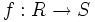# Primeness is contraction-closed

Jump to: navigation, search

This article gives the statement (and possibly proof) of a property of ideals in commutative unital rings satisfying a metaproperty of ideals in commutative unital rings

## Statement

### Property-theoretic statement

The property of ideals in commutative unital rings of being a prime ideal satisfies the metaproperty of ideals in commutative unital rings of being contraction-closed.

### Verbal statement

Given a homomorphism of commutative unital rings, the contraction of a prime ideal in the ring on the right, is a prime ideal in the ring on the left.

### Symbolic statement

Suppose$f:R \to S$ is a homomorphism of commutative unital rings. Then for any prime ideal$I$ of$S$,$I^c = f^{-1}(I)$ (called the contraction of$I$) is a prime ideal of$R$.

## Importance

This fact allows us to view the spectrum of a commutative unital ring as a contravariant functor, because it allows us to use a homomorphism of commutative unital rings$f:R \to S$ to define a backward map$Spec(f): Spec(S) \to Spec(R)$, by contraction.

## Proof

Fill this in later